Invented by the French mathematician Pierre-Simon Laplace in 1800s, Laplace is very popular in the universe of Electrical Engineering, for it has various practical applications.

So, what does it mean by “A Laplace of a function?”

Try to understand it like an operation. Just like in Calculus, we often talk about “taking log of a function”, “taking inverse of a function” etc., likewise we have a procedure to take Laplace of a function (A particular integral transform)

Given a function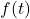defined for all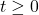, the Laplace transform ofis the functiondefined as follows:Notice that “L” like symbol? That is a symbol to represent Laplace of a function (Just like logarithm has “log”). Customarily, Laplace of a function is represented with variable “s”.

Linearity of the Laplace Transform: Ifandare constants, thenI’ve talked about deriving Laplace of various functions in the video. Nevertheless, here’s is a table of Laplace transformations of the functions used frequently.

Inverse Laplace: Just as we have Laplace, we also have Inverse Laplace.

If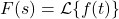, thenis called the inverse Laplace transform ofand we writeFor instance, it follows thatAlso,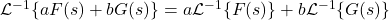Customarily, Inverse Laplace is represented with variable “t”

For inverse Laplace as well, we can refer the same table mentioned above. Just this time we need to read from right to left.

Inverse Laplace of complex functions: Remember partial fractions? The same will be used to find Inverse Laplace of certain complex functions as follows:

Example: Find the inverse Laplace transform of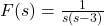Solution: We first find a partial fraction decomposition of. We have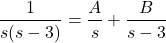Now, we just have to follow the procedure of partial fractions to find A and B, and then eventually find the inverse of these two expressions.

I have covered various examples in the video.